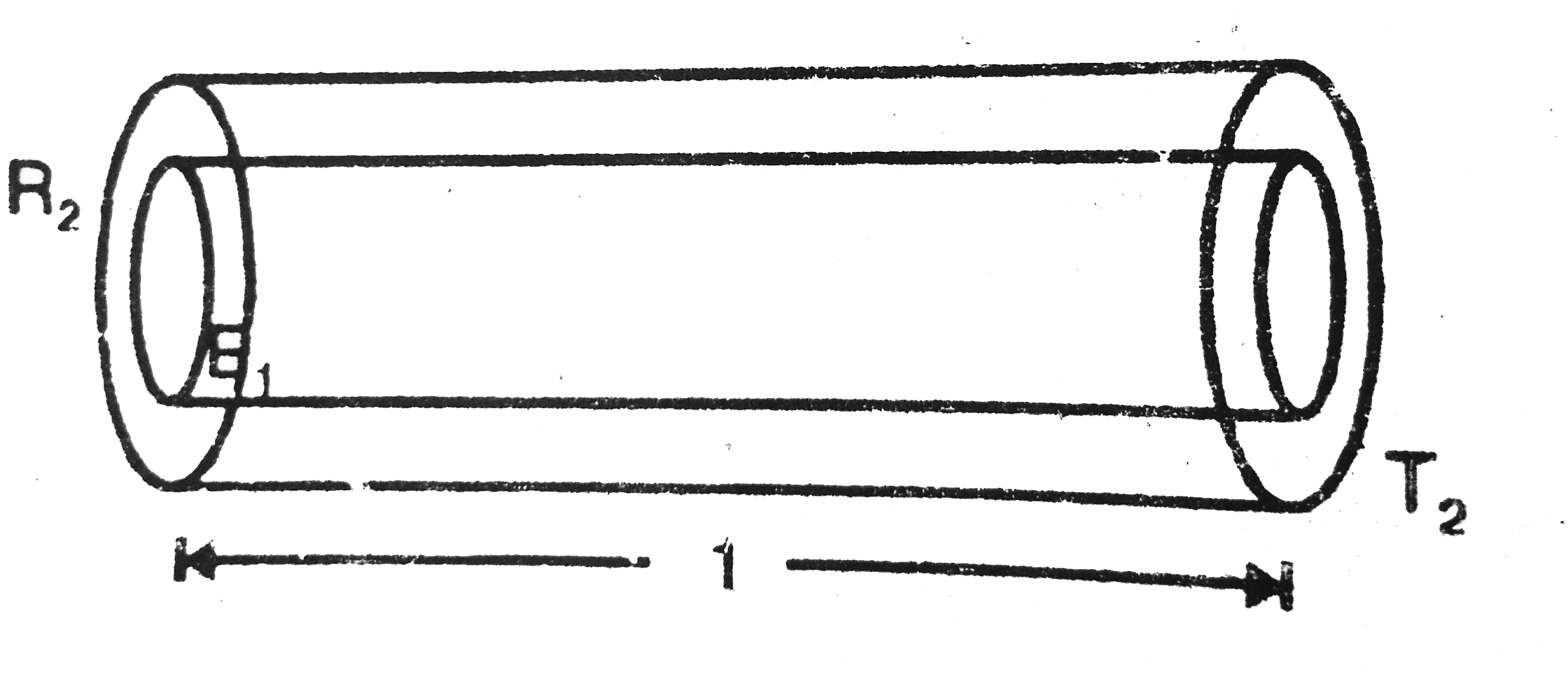# A hollow tube has a length l, inner radius R_(1) and outer radius R_(2) . The material has a thermal conductivity K. Find the heat flowing through

26 views
in Physics
closed
A hollow tube has a length l, inner radius R_(1) and outer radius R_(2) . The material has a thermal conductivity K. Find the heat flowing through the walls of the tube if (a) the flat ends are maintained at temperature T_(1) and T_(2)(T_(2)gtT_(1) (b) the inside of the tube is maintained at temperature T_(1) and the outside is maintained at T_(2) .

by (90.1k points)

T_2gtT_1
A=pi(R_2^2-R_1^2)
so, (dQ)/(dt)=(KA(T_2-T_1))/l
=(K pi(R_2^2-R_1^2)(T_2-T_1))/l
considering a concentric cylindtical cell of radius R and thickness DR.The radial heat flow through the cell.
H=(dQ)/(dt)=-KA(d theta)/(dr)
[-ve because as r increases Q decreases]
A=2 pi rl,
H=-2 pi rl K(d theta)/(dr)
or, int_(r_1)^(r_2)(dr)/r=-(2 pi rlK)/H int_(T_1)^(T_2)d theta
Integrating and simplifying we get
H=(dQ)/(dt)=(2 pi Kl(T_2-T_1))/(In(R_2//R_1)).